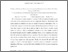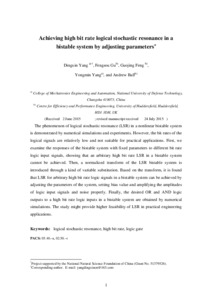Yang, Dingxin, Gu, Fengshou, Feng, Guojing, Yang, Yongmin and Ball, Andrew (2015) Achieving high bit rate logical stochastic resonance in a bistable system by adjusting parameters. Chinese Physics B, 24 (11). p. 110502. ISSN 1674-1056
Abstract

The phenomenon of logical stochastic resonance (LSR) in a nonlinear bistable system is demonstrated by numerical simulations and experiments. However, the bit rates of the logical signals are relatively low and not suitable for practical applications. First, we examine the responses of the bistable system with fixed parameters to different bit rate logic input signals, showing that an arbitrary high bit rate LSR in a bistable system cannot be achieved. Then, a normalized transform of the LSR bistable system is introduced through a kind of variable substitution. Based on the transform, it is found that LSR for arbitrary high bit rate logic signals in a bistable system can be achieved by adjusting the parameters of the system, setting bias value and amplifying the amplitudes of logic input signals and noise properly. Finally, the desired OR and AND logic outputs to a high bit rate logic inputs in a bistable system are obtained by numerical simulations. The study might provide higher feasibility of LSR in practical engineering applications.

Information
Library
DocumentsPreview
Chinese Physics 151357-En by Fgu.pdf - Accepted Version